SUPPLEMENTAL DOCUMENT SD-3

FOR PART IVC – Quality Assurance/Uncertainty

Measurement Uncertainty for Weight Determinations in Seized Drug Analysis

NOTE: Changes have been incorporated throughout Revision 2 to include a Table of Contents, an additional example of Dynamic Weighing and a more thorough discussion of correlation.

Introduction

A        Example 1: Dynamic Weighing of a Single Item Using a Budget Table

B        Example 2: Static Weighing of a Single Item Using a Budget table

C        Example 3: Static Weighing of a Single Item Using Control Chart Data in a Budget Table

D        Example 4: Static Weighing of Multiple Items to Obtain a Total Net Weight

E        References

Introduction

The following examples demonstrate the application of an uncertainty budget approach for weight determinations and should be used in conjunction with SWGDRUG Recommendations Part IV C, Section 4.  These examples are designed to assist laboratories in developing uncertainty budgets relevant to their procedures and practices.  They should not be directly applied to methodology used in laboratories without first considering the specific purpose of a method, its relevant operational environment and the operational capabilities and parameters of the balance.

It is assumed that the value being reported is the conventional mass and final results are rounded to the precision of the balance.  The calculations shown in the uncertainty budgets were done using a spreadsheet. In some intermediate calculations, additional digits are shown for illustrative purposes.  How the results are reported would depend on laboratory policy.  The term “weight” is used interchangeably with “conventional mass”, the quantity typically reported.  Definitions for the statistical terms used can be found in the SWGDRUG glossary Part IV C, Annex A or references listed below.  The references also contain additional examples and detailed information regarding estimation of uncertainty.

Weighings can be obtained using dynamic or static operations.  A dynamic weighing process involves placing a weighing vessel on a balance, taring the balance, and adding material immediately to the weighing vessel without removing it from the balance.  A static weighing process involves removal of the tared weighing vessel, filling with material, and then returning to the balance to obtain the net weight.  Examples are included in this document for both scenarios.

When the determination of a net weight requires more than one weighing event, the weighing events may be uncorrelated, correlated, or partially correlated.  In practice the correlation, measured as the correlation coefficient (r), is difficult to determine.  In the following examples, values of r have not been empirically determined. Rather, values of r have been selected to represent a conservative approach which will likely result in an overestimation of the measurement uncertainty.  Where applicable, references are provided for laboratories that elect to establish their own correlation values.

A         Example 1: Dynamic Weighing of a Single Item Using a Budget Table

Scenario: A laboratory must determine the weight of a white powder (approximately 30 g) received in a plastic bag.  The bag and its contents are considered to be one item. The decision is made to weigh the material using a two place (readability of 0.01g) balance.  The following conditions apply: the operator is competent on the use of the balance; the balance is calibrated and certified as per established laboratory protocols; the balance is being used above the defined minimum balance load; and the balance is performing within the manufacturers’ specifications.  The balance operates in a controlled environment using a draft shield with ambient temperature varying within a range of ±5 °C.

The weight is determined as follows: A weighing vessel is placed on the balance and tared.  The analyst immediately transfers the contents of the plastic bag to the tared weighing vessel without removing it from the balance and records the net weight of the material.  The entire operation is considered as a single weighing event (a dynamic weighing).

The net weight obtained for the powder is 30.03 grams.

## A.1      Factors contributing to weight measurement uncertainty

The factors considered in estimating the measurement uncertainty include readability; repeatability; linearity; buoyancy; temperature effects; uncertainty from the balance calibration report; and sample loss in transfer.  Although in some cases sample losses could be large, the inability to accurately estimate the uncertainty due to sample losses is not deemed a major concern since sample losses always result in underestimation of the quantity of a substance being weighed.  Therefore, uncertainty due to sample loss is not included in any of the uncertainty computations given in this document (SD-3).

Buoyancy is difficult to account for in seized drug cases because the density of the material being weighed must be known.  However, for material that has a lower density than the steel calibration weights (8.0 g/cm3) the bias imparted is always negative and the weight displayed by the balance will be less than the true weight of the material.  Ignoring buoyancy contributes a small systematic error that represents no more than 0.1% bias to the weight.  Therefore, buoyancy corrections are not made in any uncertainty computations shown in this document (SD-3).

Based on the current calibration and performance certification for the balance and given that the balance is operating within specifications, other factors (e.g. environmental, static electricity, corner loading) are deemed insignificant in this example.  Laboratories should examine their balances, calibration reports, methods, circumstances, and applications to determine which factors are significant and which are insignificant for their particular application.

The factors deemed significant in this example are expressed in the budget table to follow.

## A.2      Uncertainty Budget Table

 Factors Valuea Distribution Standard uncertainty (u), g Index (Relative contribution)b Readabilityc,d 0.01 g Rectangular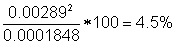Repeatability (s)e 0.010 g Normal 0.010 54.1% Linearityc,f 0.02 g Rectangular18.0% Temperature coefficientg 6 ppm/°C ( 6x10-6g/g /°C) Rectangular0.1% Uncertainty from balance calibration report (U, coverage factor k=2)h 0.0131 g Normal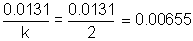23.2% Subtotal of individual u values: (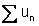): 0.02573 Sum of relative contributions: 100% Subtotal of squared u values: (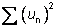): 0.0001848

## A.3      Notes for the Uncertainty Budget Table (A.2)

a.         This value could be a standard uncertainty, the range or half-width of a rectangular distribution, an expanded uncertainty, or some other quantity reported to express the level of uncertainty arising from a specified source.  The value of all factors should be in the same units.

b.This value is used to determine which factors are significant.

c.         The range of a rectangular distribution is from -a to +a.  Therefore, half the value of the full range is used to determine the standard uncertainty.

d.         Obtained from the current calibration and performance certification for the balance and assumes that the balance has a single readability range.

e.         Determined empirically in the laboratory.

f.          This value is the maximum permitted deviation across the mass range of the balance.

g.         Value obtained from manufacturer specifications is ± 5°C.  Since the distribution is rectangular, half the range is used (5°C).

h.         A conservative approach would involve the measurement of uncertainty of the balance calibration at the upper working mass range of the balance.

## A.4      Calculation of combined standard uncertainty

Considering all factors noted above (A.2) as uncorrelated for a single weighing event, the combined standard uncertainty can be expressed mathematically as: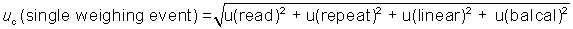where u is the standard uncertainty and uc is combined standard uncertainty.  The factor u(temperature coefficient) is not included in the combined uncertainty due to its minimal relative contribution to the total standard uncertainty.

Therefore, the combined standard uncertainty is: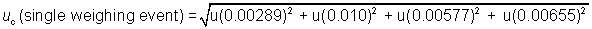Uc = 0.0136

## A.5      Calculation of expanded uncertainty

The expanded uncertainty is expressed mathematically as:

U = k*uc

Using a coverage factor k = 2 (confidence level of approximately 95%, assuming the net mass follows a normal distribution[I]):

U = 2*0.0136 g = 0.0272 g

Using a coverage factor k = 3 (confidence level of approximately 99% assuming the net mass follows a normal distribution):

U = 3*0.0136 g = 0.0408 g

## A.6.2  Net Weight: 30.03 g ± 0.04 g (k=3)

B         Example 2: Static Weighing of a Single Item Using a Budget Table

Scenario: The scenario is the same as in Example 1.

The weight is determined as follows:  A weighing vessel is placed on the balance and tared.  It is then removed from the balance and the powder is transferred to the weighing vessel, which is placed on the balance and a reading obtained (a static weighing).

The net weight obtained for the powder is 30.03 grams.

# B.1       Factors contributing to weight measurement uncertainty

The factors are the same as in Example 1.

# B.2      Uncertainty Budget Table

The uncertainty budget table is the same as in Example 1. The factor u(temperature coefficient) is not included in the combined uncertainty due to its minimal relative contribution to the total standard uncertainty.

# B.3      Calculation of combined standard uncertainty

The combined standard uncertainty for a single weighing event is the same as in Example 1:uc = 0.0136

In this case, the calculation of total uncertainty for the net mass is: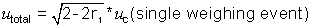where r1 is the correlation coefficient between the weighing associated with the tare and the weighing of the material.  Because this is a static weighing process, two separate weighing events are considered-the taring of the weigh vessel and the weighing of the material.

In practice, the value of r1 is difficult to determine[II]. In this example, the most conservative approach is to assume that the two weighing events are completely negatively correlated.  As a result, r1 is assigned the value of -1. This will likely result in overestimation of the uncertainty.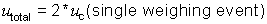utotal = 2 * 0.0136 g = 0.0272 g

Alternatively, the laboratory may elect to empirically determine another value for r1[III].

# B.4      Calculation of expanded uncertainty

The expanded uncertainty is expressed mathematically as:

U = k*utotal

Using a coverage factor k = 2 (confidence level of approximately 95%, assuming the net mass follows a normal distribution):

U = 2*0.0272 g = 0.0544 g

Using a coverage factor k = 3 (confidence level of approximately 99% assuming the net mass follows a normal distribution):

U = 3*0.0272 g = 0.0816 g

# B.5  Results

B.5.1 Net Weight: 30.03 g ± 0.05 g (k=2)

B.5.2 Net Weight: 30.03 g ± 0.08 g (k=3)

Scenario: In this example, the measurement uncertainty is calculated using control chart data obtained from a measurement quality assurance process that mimics casework samples as closely as possible. All other conditions and assumptions are the same as Example 2, including the use of a static weighing process.

The control chart should capture uncertainty deemed appropriate to the specific laboratory and procedure and could include factors such as environmental conditions, analysts, and sample types.  A conservative approach is to select the largest standard deviation if a range of masses is charted.

# C.1       Factors contributing to weight measurement uncertainty

As the laboratory’s control chart is well established, it is expected to capture all of the factors described in Example 1 except linearity and balance calibration uncertainty.

# C.2      Uncertainty Budget Table

 Factors Value Distribution Standard uncertainty (u), g Index (Relative contribution) Control chart standard deviation (s)a 0.0313 g Normal 0.0313 92.8 % Linearityb 0.02 g Rectangular3.2 % Uncertainty from balance calibration report (U, coverage factor k=2) 0.0131 g Normal4.1 % Subtotal of individual u values: (): 0.0436 Sum of relative contributions: 100% Subtotal of squared u values: (): 0.00106

# C.3      Notes for the Uncertainty Budget Table (C.2)

a.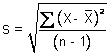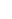b. This value is the maximum permitted deviation across the mass range of the balance.

# C.4      Calculation of combined standard uncertainty

The combined standard uncertainty per weighing event can be expressed in this example mathematically as: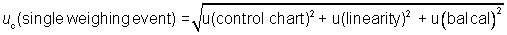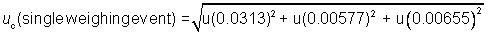Under the assumption about correlation between the sample and tare weighings made in Example 2, the total uncertainty is: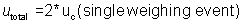utotal = 2 * 0.0325 g = 0.0650 g

# C.5      Calculation of expanded uncertainty

The expanded uncertainty is expressed mathematically as:

U = k*utotal

Using a coverage factor k = 2 (confidence level of approximately 95% assuming the net mass follows a normal distribution):

U = 2*0.0650 g = 0.130 g

Using a coverage factor k = 3 (confidence level of approximately 99% assuming the net mass follows a normal distribution 2):

U = 3*0.0650 g = 0.195 g

# C.6  Results

C.6.1 Net Weight: 30.03 g ± 0.13 g (k=2)

C.6.2 Net Weight: 30.03 g ± 0.20 g (k=3)

Scenario: In this example, the laboratory must determine the net weight of a white powder, received in 15 similar plastic bags (15 items), which appear to weigh approximately 30 g each.  All other conditions are the same as Example 3.

The net weight of the contents of each bag was determined as in Examples 2 and 3 (a static weighing procedure). The total net weight obtained for the powder, determined by individually placing the material from each plastic bag inside 15 separate tared weighing vessels, is 458.37 grams.

# D.1       Factors contributing to weight measurement uncertainty

Same as in Example 3.  The laboratory could also choose to use the uncertainty budget approach as presented in Example 2.

# D.2       Uncertainty Budget Table

Same as Example 3.  The laboratory could also choose to use the uncertainty budget as presented in Example 2.

# D.3      Calculation of combined standard uncertainty

Using the correlation between weighing of sample and tare assumed in Examples 2 and 3, the calculation of total uncertainty for the net mass of the material from all 15 bags is: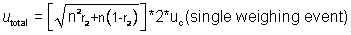where r2 is a correlation coefficient describing the relationship between the weighings of any pair of bags.  Note this correlation (r2) is not the same correlation as between the tare and the weighing (r1).

In practice, the value of r2 is difficult to determine[IV].  In this example, the most conservative approach is to assume that the weighings between any two bags are all completely positively correlated.  As a result, r2 is assigned the value of +1.  This will likely result in overestimation of the uncertainty.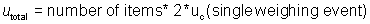utotal = 15 items * 2 * 0.0325 g = 0.975 g

Alternatively, the laboratory may elect to empirically determine another value for r2[V].

# D.4      Calculation of expanded uncertainty

The expanded uncertainty per weighing event (U) is expressed mathematically as:

U = k*utotal

Using a coverage factor k = 2 (confidence level of approximately 95%):

U = 2*0.975 g = 1.95 g

Using a coverage factor k = 3 (confidence level of approximately 99%):

U = 3*0.975 g = 2.93 g

# D.5  Results

D.5.1 Net Weight: 458.37 g ± 1.95 g (k=2)

D.5.2  Net Weight: 458.37 g ± 2.93 g (k=3)

# E.1      Books

E.1.1    Dieck, R.H. 2007. Measurement Uncertainty: Methods and Applications. 4th ed. Research Triangle Park, NC: The Instruments, Systems, and Automation Society (ISA).

E.1.2    Jones, F. E., and R. M. Schoonover. 2002. Handbook of Mass Measurement. Boca Raton, FL: CRC Press.

E.1.3     Kimothi, S.K. 2002. The Uncertainty of Measurements: Physical and Chemical Metrology: Impact and Analysis. Milwaukee, WI: ASQ Press (American Society for Quality).

E.1.4    Kirkup, L., and R. B. Frenkel. 2006. An Introduction to Uncertainty in Measurement. Cambridge, UK: Cambridge University Press.

E.1.5    Lawn, R., and E. Pritchard. 2003. Measurement of Mass, Practical Laboratory Skills Training Guides Series: Royal Society of Chemistry.

E.1.6    Pavese, F., and A.B. Forbes, eds. 2009. Data Modeling for Metrology and Testing in Measurement Science. Edited by N. Bellomo, Modeling and Simulation in Science, Engineering, and Technology. Boston: Birkhauser.

E.1.7    Taylor, J.R. 1997. An Introduction to Error Analysis: The Study of Uncertainties in Physical Measurements (2nd ed). Sausalito, CA: University Science Books.

# E.2      Journal articles and reviews

E.2.1    Bremser, W., J. Viallon, and R. I. Wielgosz. 2006. Influence of correlation on the assessment of measurement result compatibility over a dynamic range. Metrologia 44(6): 495-504.

E.2.2    Brown, R. J. C., and M. J. T. Milton. 2007. Developments in accurate and traceable chemical measurements. Chemical Society Reviews 36 (6):904-913.

E.2.3    Burns, M. 2004. Current practice in the assessment and control of measurement uncertainty in bio-analytical chemistry. Trac-Trends in Analytical Chemistry 23(5):393-398.

E.2.4    Choi, J., E. Hwang, H. Y. So, and B. Kim. 2003. An uncertainty evaluation for multiple measurements by GUM. Accreditation and Quality Assurance 8(1): 13-15.

E.2.5    Ellison, S. L. R. 2005. Including correlation effects in an improved spreadsheet calculation of combined standard uncertainties. Accreditation and Quality Assurance 10(7):338-43.

E.2.6      Gonzalez, A. G., and M. A. Herrador. 2007. The assessment of electronic balances for accuracy of mass measurements in the analytical laboratory. Accreditation and Quality Assurance 12(1):21-29.

E.2.7    Haesselbarth, W., and W. Bremser. 2004. Correlation between Repeated Measurements: Bane and Boon of the GUM Approach to the Uncertainty of Measurement. Accreditation and Quality Assurance 9(10): 597-600.

E.2.8    Hasselbarth, W., and W. Bremser. 2007. Measurement uncertainty for multiple measurands: characterization and comparison of uncertainty matrices. Metrologia 44(2):128-45.

E.2.9    Kehl, K. G., K. Weirauch, S. Wunderli, and V. R. Meyer. 2000. The influence of variations in atmospheric pressure on the uncertainty budget of weighing results. Analyst 125(5):959-962.

E.2.10  Kessel, R., M. Berglund, P. Taylor, and R Wellum. 2001. How to treat correlation in the uncertainty budget, when combining results from different measurements, in Advanced Mathematical and Computational Tools in Metrology V, edited by P. Ciarlini, M.G. Cox, E. Filipe, F. Paveses and D. Richter. Series on Advances in Mathematics for Applied Sciences - Vol. 57. Singapore: World Scientific Publishing, 2001, p. 231-241.

E.2.11  Kessel, R., R. Kacker, and M. Berglund. 2006. Coefficient of contribution to the combined standard uncertainty. Metrologia 43(4): S189-S95.

E.2.12  Konieczka, P. 2007. The role of and the place of method validation in the quality assurance and quality control (QA/QC) system. Critical Reviews in Analytical Chemistry 37(3):173-190.

E.2.13  Linsinger, T. P. J. 2008. Use of recovery and bias information in analytical chemistry and estimation of its uncertainty contribution. Trac-Trends in Analytical Chemistry 27 (10):916-923.

E.2.14  Meyer, V. R. 2007. Measurement uncertainty. Journal of Chromatography A 1158 (1-2):15-24.

E.2.15  Populaire, S., and E. C. Gimenez. 2006. A simplified approach to the estimation of analytical measurement uncertainty. Accreditation and Quality Assurance 10 (9):485-493.

E.2.16  Pozivil, M., W. Winiger, S. Wunderli, and V. R. Meyer. 2006. The influence of climate conditions on weighing results. Microchimica Acta 154 (1-2):55-63.

E.2.17  Rios, A., and M. Valcarcel. 1998. A view of uncertainty at the bench analytical level. Accreditation and Quality Assurance 3 (1):14-19.

E.2.18  Schoonover, R. M., and F. E. Jones. 1981. Air Buoyancy Correction in High-Accuracy Weighing on Analytical Balances. Analytical Chemistry 53 (6):900-902.

E.2.19  Wunderli, S., G. Fortunato, A. Reichmuth, and P. Richard. 2003. Uncertainty evaluation of mass values determined by electronic balances in analytical chemistry: a new method to correct for air buoyancy. Analytical and Bioanalytical Chemistry 376 (3):384-391.

# E.3      On-line resources

E.3.1    EURACHEM. Quantifying Uncertainty in Analytical Measurement 2007. Available from http://www.measurementuncertainty.org/index.html.

E.3.2    JCGM. JCGM 100-2008: Evaluation of measurement data (GUM 1995 with minor corrections). JCGM: Joint Committee for Guides in Metrology, 2008 2008. Available from http://www.iso.org/sites/JCGM/GUM/JCGM100/C045315e-html/C045315e.html?csnumber=50461.

E.3.3    NIST. 2006. NIST/SEMATECH e-Handbook of Statistical Methods: National Institute of Science and Technology, U.S. Department of Commerce. Available from http://www.itl.nist.gov/div898/handbook.

E.3.4    NIST. 2000. The NIST Reference on Constants, Units, and Uncertainty. Available from http://physics.nist.gov/cuu/Uncertainty/index.html.

E.3.5    NIST 1994. NIST Technical note 1297 Guidelines for Evaluating and Expressing the Uncertainty of NIST Measurement Results. National Institute of Science and Technology, U.S. Department of Commerce. Available from http://physics.nist.gov/Pubs/guidelines/contents.html.

E.3.6    SIM Guidelines on the calibration of non-automatic weighing instruments. 2009.  SIM MWG7/cg-01/v.00. (Identical to the original EURAMET/cg-18/v.02) Available from http://www.dkd.eu/dokumente/Richtlinien/EURAMET_cg-18_v.02.pdf.

End of Document

[I] The approximate confidence levels given in this document (SD-3) assume that the quantities for which expanded uncertainties are being computed each approximately follow a normal distribution.  If this assumption does not hold, the actual confidence level attained for these uncertainty intervals may be lower or higher than the desired levels of 95% or 99%

[II] The theoretical limits of r1 are +1 and -1.  In reality, r1 will have a value between these two limits.  In lieu of the laboratory determining the value of r1 empirically, assigning r1 a value of -1 is the most conservative approach.  These limits can be derived from the law of propagation of uncertainty which is described in book references E.1.1 (Dieck, Unit 5); E.1.3 (Kimothi, p. 205-210); and E.1.7 (Taylor, Chapter 3).

[III] If not known from relevant references in the metrology literature or experience, the value of the correlation coefficient r1 may be determined empirically by performing an experiment with mass standards that approximate the gross and tare masses of the seized drugs on the balance.  If the correlation between the gross and tare masses is based on common corrections applied in the computation of each mass, the value of the correlation coefficient r1 can be obtained using the methods in Section F.1.2.3 of the GUM[D.3.2].  Several articles addressing correlation are included in Section E.2.

[IV] In this situation where multiple weighings are added to obtain a total weight, the theoretical limits of r2 are 0 and +1.  In reality, r2 will have a value between these two limits.  Unlike r1, the value of r2 cannot be negative because two positive values are added to obtain it.  These limits can be derived from the law of propagation of uncertainty which is described in book references E.1.1 (Dieck, Unit 5); E.1.3 (Kimothi, p. 205-210); and E.1.7 (Taylor, Chapter 3). In lieu of the laboratory determining the value of r2 empirically, assigning r2 a value of 1 is the most conservative approach.  This also arises from the derivation of the law of propagation of uncertainty. Several articles addressing correlation are included in Section E.2.

[V] If not known from relevant references in the metrology literature or experience, the value of the correlation coefficient r2 may be determined empirically by performing an experiment with measurement assurance standards that approximate composition and masses of the bags in the case. If the correlation between masses of different bags is based on common corrections applied in the computation of each mass, the value of the correlation coefficient r2 can be obtained using the methods in Section F.1.2.3 of the GUM [E.3.2]. Several articles discussing correlation are included in Section E.2.